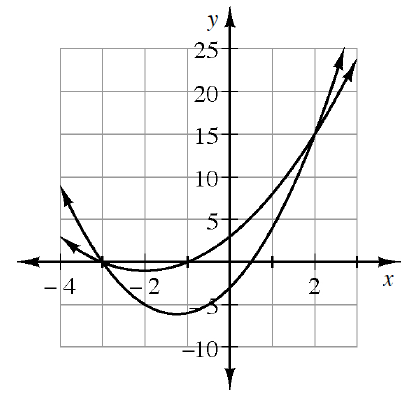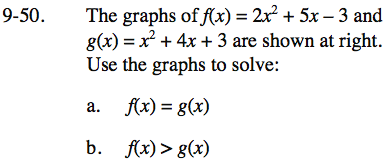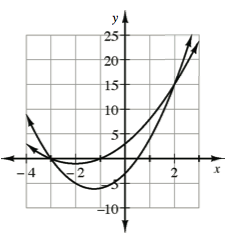### Home > INT3 > Chapter Ch9 > Lesson 9.1.4 > Problem9-50

9-50.
1.The graphs of f(x) = 2x2 + 5x – 3 and g(x) = x2 + 4x + 3 are shown at right. Use the graphs to solve: Homework Help ✎

1. f(x) = g(x)

2. f(x) > g(x)When the equations of graphs are set equal to each other, their points overlap.In terms of x, where on the graph do the y-values of f(x) exceed those of g(x)?

x < −3 and x > 2